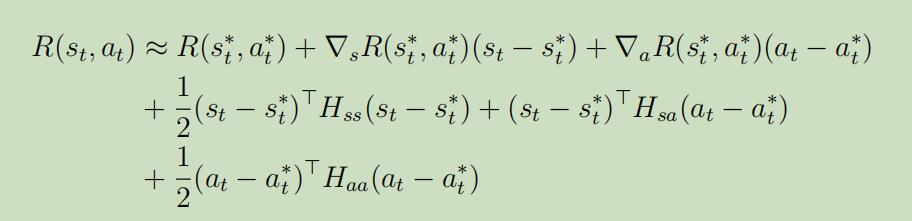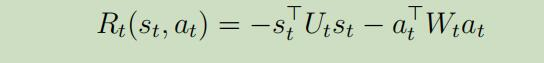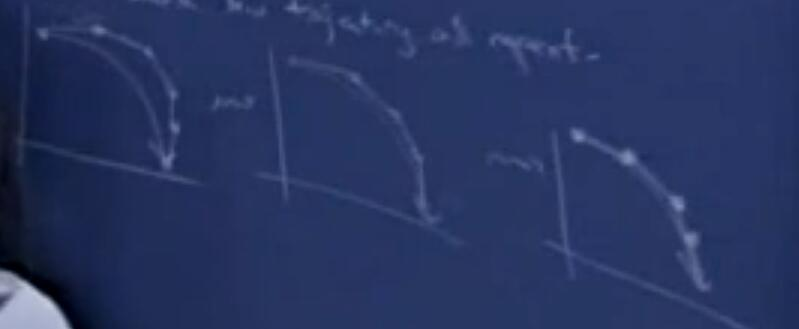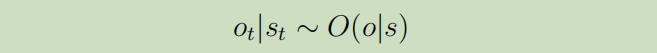# 吴恩达Stanford机器学习公开课（十九）笔记

## Lecture 19 - From non-linear dynamics to LQR，Linear Quadratic Gaussian (LQG)

Posted by Yunlongs on July 13, 2019

# Lecture 19 - From non-linear dynamics to LQR，Linear Quadratic Gaussian (LQG)

## 1. From non-linear dynamics to LQR

### 1.2 微分动力规划（Differential Dynamic Programming (DDP)）

Note： 我们可以对反馈$R^{(t)}$做相似的二阶泰勒扩展处理：可以被重写为：Note： 如果LQR的轨道与线性化估计的轨道差的太远，将会产生很多问题，但是可以通过重塑反馈函数来解决。## 2. 线性二次高斯（Linear Quadratic Gaussian (LQG)）

POMDP是在MDP上增加了一个额外的观察层，所以我们引入了一个新的变量$o_t$，它服从条件分布:因为协方差矩阵会因为时间而越来越大，计算起来就越来越困难，所以我们将使用Kalman Filter算法来计算Remark： 如果我们细心的话，我们可以注意到我们并不需要时间t之前的观察！更新步仅依赖于前面的分布，当把上面所有东西放在一起后，算法首先正向计算$K_{t}, \Sigma_{t | t}$ 和 $s_{t | t}$。然后在反向计算这些量$\Psi_{t}, \Psi_{t}$ and $L_{t}$。最后，最优政策为$a_{t}^{*}=L_{t} s_{t | t}$。